# PRAM Parallel Random Access Machine Sharedmemory multiprocessor unlimited

• Slides: 18PRAM • Parallel Random Access Machine • Shared-memory multiprocessor • unlimited number of processors, each – has unlimited local memory – knows its ID – able to access the shared memory • unlimited shared memory Slide 1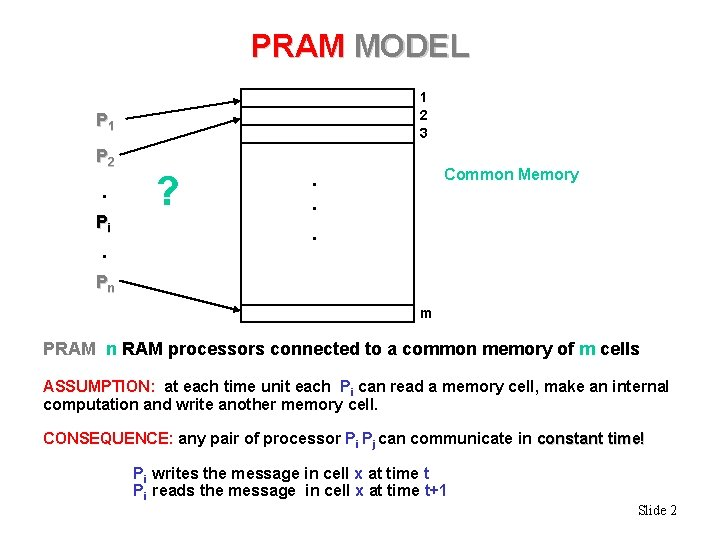PRAM MODEL 1 2 3 P 1 P 2 . Pi . ? Common Memory . . . Pn m PRAM n RAM processors connected to a common memory of m cells ASSUMPTION: at each time unit each Pi can read a memory cell, make an internal computation and write another memory cell. CONSEQUENCE: any pair of processor Pi Pj can communicate in constant time! Pi writes the message in cell x at time t Pi reads the message in cell x at time t+1 Slide 2PRAM • Inputs/Outputs are placed in the shared memory (designated address) • Memory cell stores an arbitrarily large integer • Each instruction takes unit time • Instructions are synchronized across the processors Slide 3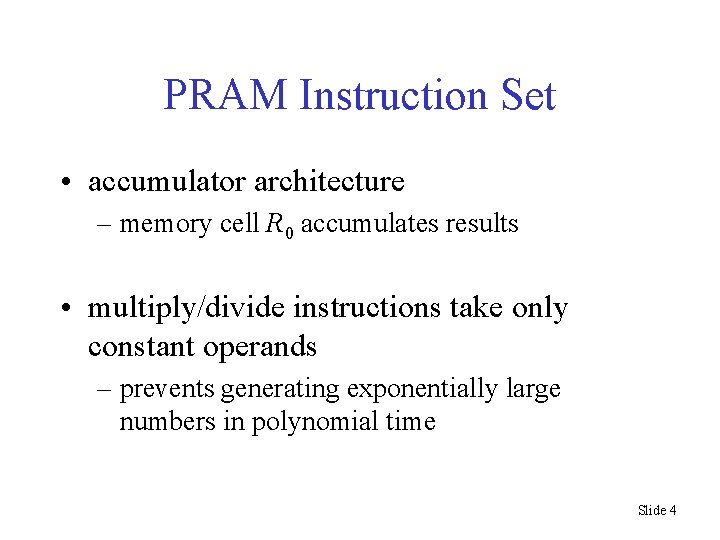PRAM Instruction Set • accumulator architecture – memory cell R 0 accumulates results • multiply/divide instructions take only constant operands – prevents generating exponentially large numbers in polynomial time Slide 4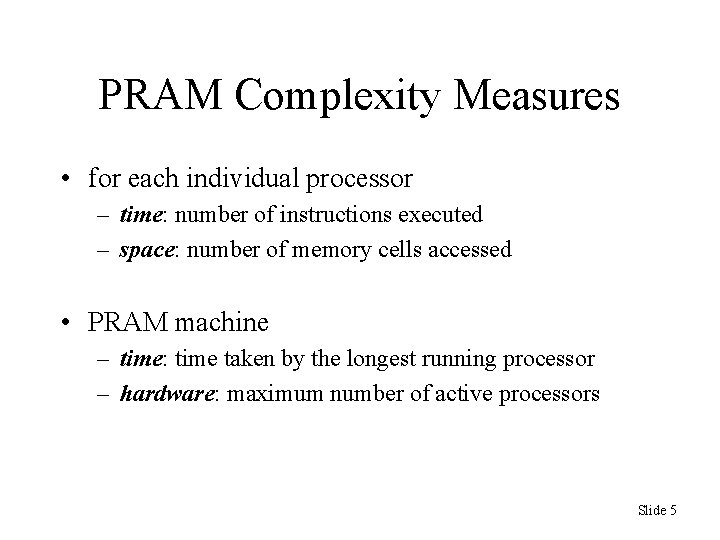PRAM Complexity Measures • for each individual processor – time: number of instructions executed – space: number of memory cells accessed • PRAM machine – time: time taken by the longest running processor – hardware: maximum number of active processors Slide 5Two Technical Issues for PRAM • How processors are activated • How shared memory is accessed Slide 6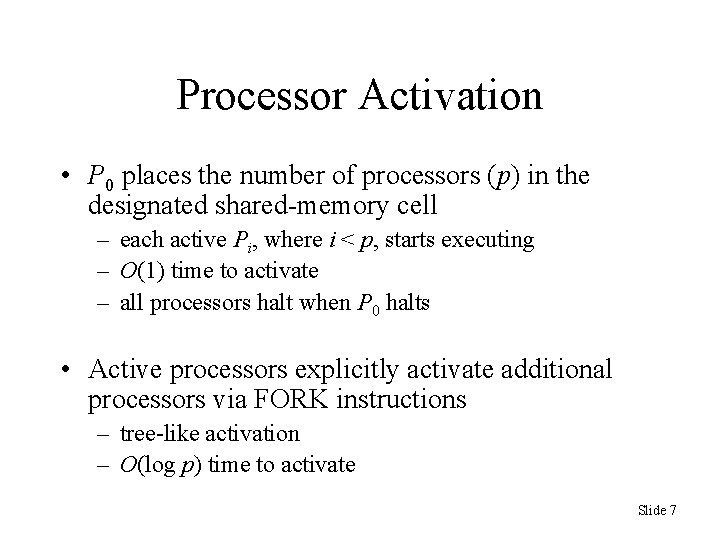Processor Activation • P 0 places the number of processors (p) in the designated shared-memory cell – each active Pi, where i < p, starts executing – O(1) time to activate – all processors halt when P 0 halts • Active processors explicitly activate additional processors via FORK instructions – tree-like activation – O(log p) time to activate Slide 7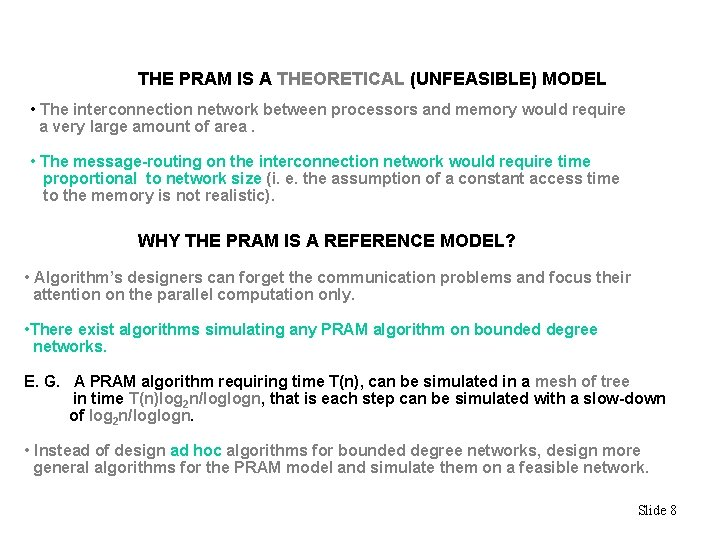THE PRAM IS A THEORETICAL (UNFEASIBLE) MODEL • The interconnection network between processors and memory would require a very large amount of area. • The message-routing on the interconnection network would require time proportional to network size (i. e. the assumption of a constant access time to the memory is not realistic). WHY THE PRAM IS A REFERENCE MODEL? • Algorithm’s designers can forget the communication problems and focus their attention on the parallel computation only. • There exist algorithms simulating any PRAM algorithm on bounded degree networks. E. G. A PRAM algorithm requiring time T(n), can be simulated in a mesh of tree in time T(n)log 2 n/loglogn, that is each step can be simulated with a slow-down of log 2 n/loglogn. • Instead of design ad hoc algorithms for bounded degree networks, design more general algorithms for the PRAM model and simulate them on a feasible network. Slide 8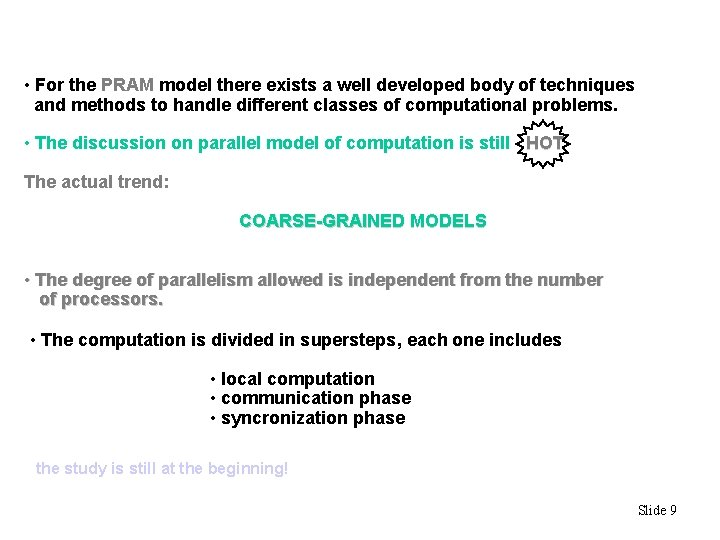• For the PRAM model there exists a well developed body of techniques and methods to handle different classes of computational problems. • The discussion on parallel model of computation is still HOT The actual trend: COARSE-GRAINED MODELS • The degree of parallelism allowed is independent from the number of processors. • The computation is divided in supersteps, each one includes • local computation • communication phase • syncronization phase the study is still at the beginning! Slide 9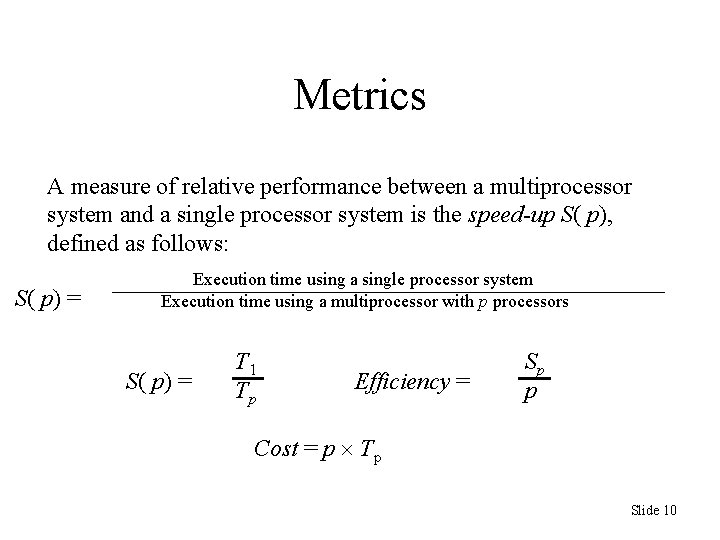Metrics A measure of relative performance between a multiprocessor system and a single processor system is the speed-up S( p), defined as follows: S( p) = Execution time using a single processor system Execution time using a multiprocessor with p processors S( p) = T 1 Tp Efficiency = Sp p Cost = p Tp Slide 10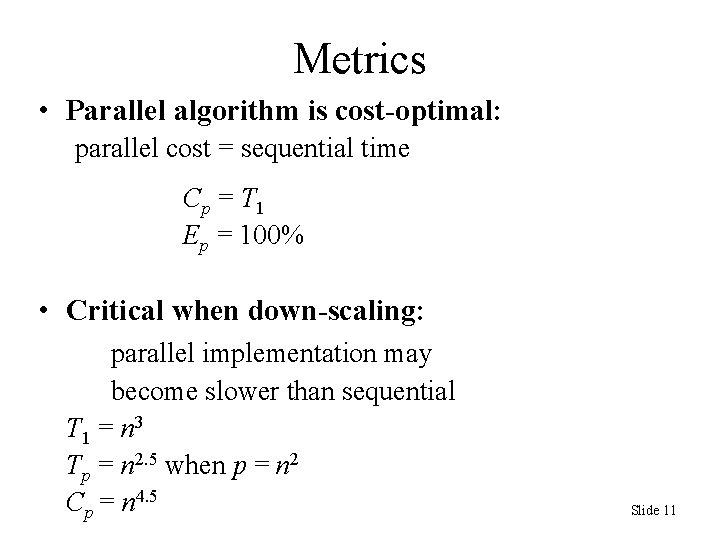Metrics • Parallel algorithm is cost-optimal: parallel cost = sequential time C p = T 1 Ep = 100% • Critical when down-scaling: parallel implementation may become slower than sequential T 1 = n 3 Tp = n 2. 5 when p = n 2 Cp = n 4. 5 Slide 11Amdahl’s Law • f = fraction of the problem that’s inherently sequential (1 – f) = fraction that’s parallel • Parallel time Tp: • Speedup with p processors: Slide 12Amdahl’s Law • Upper bound on speedup (p = ) Converges to 0 • Example: f = 2% S = 1 / 0. 02 = 50 Slide 13PRAM • Too many interconnections gives problems with synchronization • However it is the best conceptual model for designing efficient parallel algorithms – due to simplicity and possibility of simulating efficiently PRAM algorithms on more realistic parallel architectures Slide 14Shared-Memory Access Concurrent (C) means, many processors can do the operation simultaneously in the same memory Exclusive (E) not concurent • • EREW (Exclusive Read Exclusive Write) CREW (Concurrent Read Exclusive Write) – Many processors can read simultaneously a same location, but only one can attempt to write to a given location • • ERCW CRCW – Many processors can write/read at/from the same memory location Slide 15Example CRCW-PRAM • Initially – table A contains values 0 and 1 – output contains value 0 • The program computes the “Boolean OR” of A, A, A, A, A Slide 16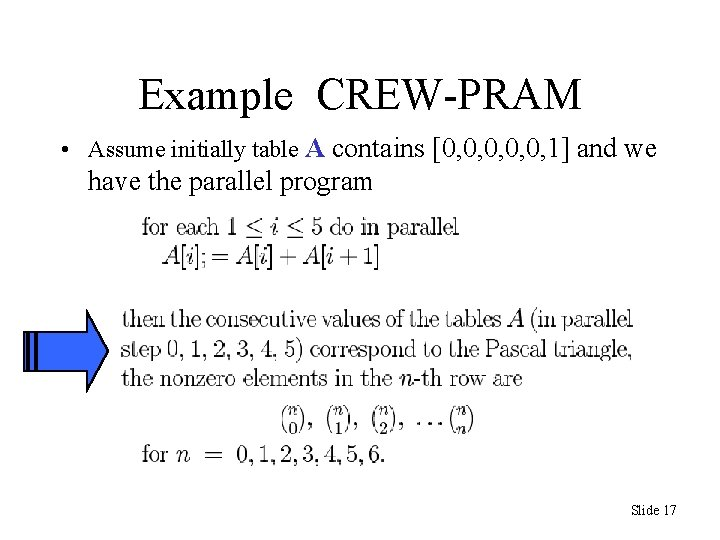Example CREW-PRAM • Assume initially table A contains [0, 0, 0, 1] and we have the parallel program Slide 17Pascal triangle PRAM CREW Slide 18